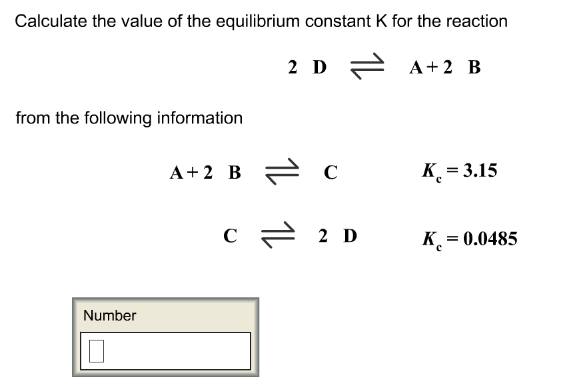# Problem: Calculate the value of the equilibrium constant K for the reaction 2D ⇌ A + 2B from the following information A + 2B ⇌ C             Kc = 3.15C ⇌ 2D                   Kc = 0.0485

###### FREE Expert Solution
89% (459 ratings)###### Problem Details

Calculate the value of the equilibrium constant K for the reaction

2D ⇌ A + 2B

from the following information

A + 2B ⇌ C             Kc = 3.15

C ⇌ 2D                   Kc = 0.0485